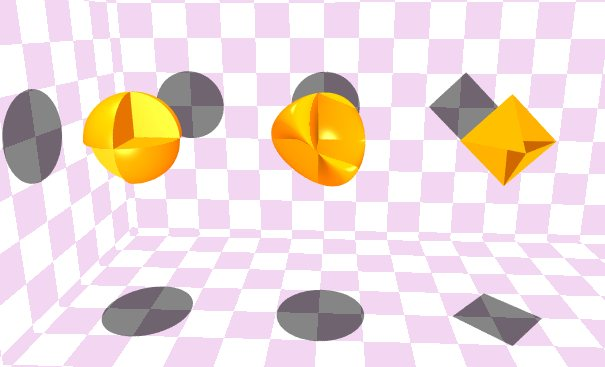Three ThingsFour out of Eight Octants in a Sphere, radius 1/2: Exact Area = 5 pi / 4 Approximate Area = 3.9267 Exact Volume = pi / 12 Approximate Volume = 0.2618 A Roman surface, x2y2+x2z2+y2z2-xyz = 0: Exact Area = ???? Approximate Area = 3.195 Exact Volume = 1 / 6 Approximate Volume = 0.1666 Four out of Eight Octants in an Octahedron (distance from center to vertex = 1/2): Exact Area = (sqrt(3) + 3) / 2 Approximate Area = 2.366 Exact Volume = 1 / 12 Approximate Volume = 0.08333

Back to the graphics gallery.